Tutorials
r programming
+1

# Logistic Regression in R Tutorial

Discover all about logistic regression: how it differs from linear regression, how to fit and evaluate these models it in R with the glm() function and more!Logistic regression is yet another technique borrowed by machine learning from the field of statistics. It's a powerful statistical way of modeling a binomial outcome with one or more explanatory variables. It measures the relationship between the categorical dependent variable and one or more independent variables by estimating probabilities using a logistic function, which is the cumulative logistic distribution.

This R tutorial will guide you through a simple execution of logistic regression:

• You'll first explore the theory behind logistic regression: you'll learn more about the differences with linear regression and what the logistic regression model looks like. You'll also discover multinomial and ordinal logistic regression.
• Next, you'll tackle logistic regresssion in R: you'll not only explore a data set, but you'll also fit the logistic regression models using the powerful glm() function in R, evaluate the results and solve overfitting.

Tip: if you're interested in taking your skills with linear regression to the next level, consider also DataCamp's Multiple and Logistic Regression course!

## Regression Analysis: Introduction

As the name already indicates, logistic regression is a regression analysis technique. Regression analysis is a set of statistical processes that you can use to estimate the relationships among variables. More specifically, you use this set of techniques to model and analyze the relationship between a dependent variable and one or more independent variables. Regression analysis helps you to understand how the typical value of the dependent variable changes when one of the independent variables is adjusted and others are held fixed.

As you already read, there are various regression techniques. You can distinguish them by looking at three aspects: the number of independent variables, the type of dependent variables and the shape of regression line.

### Linear Regression

Linear regression is one of the most widely known modeling techniques. It allows you, in short, to use a linear relationship to predict the (average) numerical value of $Y$ for a given value of $X$ with a straight line. This line is called the "regression line".

As a consequence, the linear regression model is $y= ax + b$. The model assumes that the response variable $y$ is quantitative. However, in many situations, the response variable is qualitative or, in other words, categorical. For example, gender is qualitative, taking on values male or female.

Prediciting a qualitative response for an observation can be referred to as classifying that observation, since it involves assigning the observation to a category, or class. On the other hand, the methods that are often used for classification first predict the probability of each of the categories of a qualitative variable, as the basis for making the classification.

Linear regression is not capable of predicting probability. If you use linear regression to model a binary response variable, for example, the resulting model may not restrict the predicted Y values within 0 and 1. Here's where logistic regression comes into play, where you get a probaiblity score that reflects the probability of the occurrence at the event.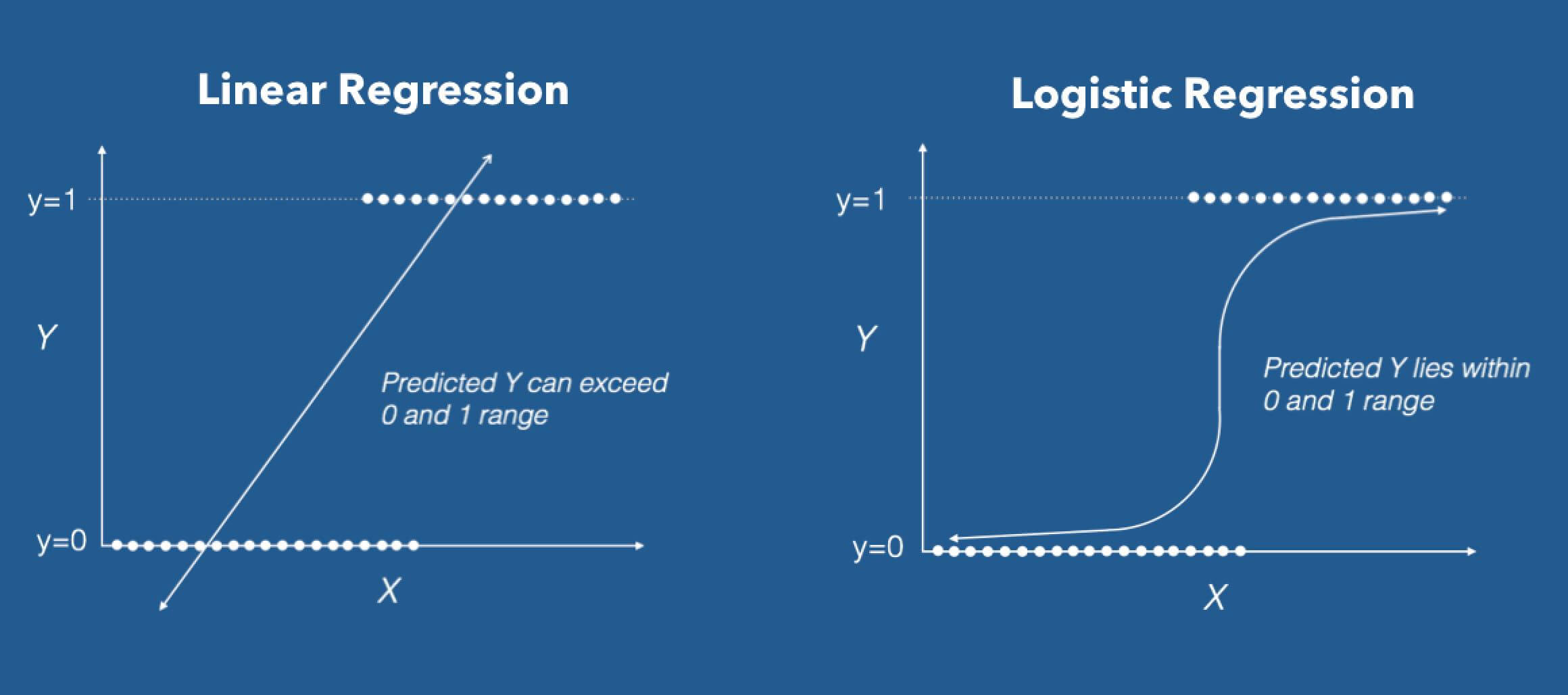### Logistic Regression

Logistic regression is an instance of classification technique that you can use to predict a qualitative response. More specifically, logistic regression models the probability that $gender$ belongs to a particular category.

That means that, if you are trying to do gender classification, where the response $gender$ falls into one of the two categories, male or female, you'll use logistic regression models to estimate the probability that $gender$ belongs to a particular category.

For example, the probability of $gender$ given $longhair$ can be written as:

$Pr(gender = female | longhair)$

The values of $Pr(gender = female | longhair)$ (abbreviated as $p(longhair)$) will range between 0 and 1. Then, for any given value of $long hair$, a prediction can be made for $gender$.

Given $X$ as the explanatory variable and $Y$ as the response variable, how should you then model the relationship between $p(X)=Pr(Y=1|X)$ and $X$? The linear regression model represents these probabilities as:

p(X)=β0 + β1X

The problem with this approach is that, any time a straight line is fit to a binary response that is coded as $0$ or $1$, in principle we can always predict $p(X) < 0$ for some values of $X$ and $p(X) > 1$ for others.

To avoid this problem, you can use the logistic function to model $p(X)$ that gives outputs between $0$ and $1$ for all values of $X$:

$$p(X) = \frac{ e^{\beta_{0} + \beta_{1}X} }{1 + e^{\beta_{0} + \beta_{1}X} }$$

The logistic function will always produce an S-shaped curve, so regardless of the value of $X$, we will obtain a sensible prediction.

The above equation can also be reframed as:

$$\frac{p(X)}{1 - p(X)} = e^{\beta_{0} + \beta_{1}X}$$

The quantity $$\frac{p(X)}{1 - p(X)}$$ is called the odds ratio, and can take on any value between $0$ and $\infty$. Values of the odds ratio close to $0$ and $\infty$ indicate very low and very high probabilities of $p(X)$, respectively.

By taking the logarithm of both sides from the equation above, you get:

$$log(\frac{p(X)}{1 - p(X)}) = \beta_{0} + \beta_{1}X$$

The left-hand side is called the logit. In a logistic regression model, increasing X by one unit changes the logit by β0. The amount that p(X) changes due to a one-unit change in X will depend on the current value of X. But regardless of the value of X, if β1 is positive then increasing X will be associated with increasing p(X), and if β1 is negative then increasing X will be associated with decreasing p(X).

The coefficients β0 and β1 are unknown, and must be estimated based on the available training data. For logistic regression, you can use maximum likelihood, a powerful statistical technique. Let's refer back to your gender classification example.

You seek estimates for β0 and β1 such that plugging these estimates into the model for p(X) yields a number close to 1 for all individuals who are female, and a number close to 0 for all individuals who are not.

This intuition can be formalized using a mathematical equation called a likelihood function:

l(β0, β1)=p(X)(1 − p(X))

The estimates β0 and β1 are chosen to maximize this likelihood function. Once the coefficients have been estimated, you can simply compute the probability of being $female$ given any instance of having $long hair$. Overall, maximum likelihood is a very good approach to fit non-linear models.

### Multinomial Logistic Regression

So far, this tutorial has only focused on Binomial Logistic Regression, since you were classifying instances as male or female. Multinomial Logistic Regression model is a simple extension of the binomial logistic regression model, which you use when the exploratory variable has more than two nominal (unordered) categories.

In multinomial logistic regression, the exploratory variable is dummy coded into multiple 1/0 variables. There is a variable for all categories but one, so if there are M categories, there will be $M−1$ dummy variables. Each category’s dummy variable has a value of 1 for its category and a 0 for all others. One category, the reference category, doesn’t need its own dummy variable, as it is uniquely identified by all the other variables being 0.

The mulitnomial logistic regression then estimates a separate binary logistic regression model for each of those dummy variables. The result is $M−1$ binary logistic regression models. Each model conveys the effect of predictors on the probability of success in that category, in comparison to the reference category.

### Ordinal Logistic Regression

Next to multinomial logistic regression, you also have ordinal logistic regression, which is another extension of binomial logistics regression. Ordinal regression is used to predict the dependent variable with ‘ordered’ multiple categories and independent variables. You already see this coming back in the name of this type of logistic regression, since "ordinal" means "order of the categories".

In other words, it is used to facilitate the interaction of dependent variables (having multiple ordered levels) with one or more independent variables.

For example, you are doing customer interviews to evaluate their satisfaction towards our newly released product. You are tasked to ask a question to respondent where their answer lies between $Satisfactory$ or $Unsatisfactory$. To generalize the answers well, you add levels to your responses such as $Very Unsatisfactory$, $Unsatisfactory$, $Neutral$, $Satisfactory$, $Very Satisfactory$. This helped you to observe a natural order in the categories.

## Logistic Regression in R with glm

In this section, you'll study an example of a binary logistic regression, which you'll tackle with the ISLR package, which will provide you with the data set, and the glm() function, which is generally used to fit generalized linear models, will be used to fit the logistic regression model.

The first thing to do is to install and load the ISLR package, which has all the datasets you're going to use.

require(ISLR)

## Warning: package 'ISLR' was built under R version 3.3.2


For this tutorial, you're going to work with the Smarket dataset within RStudio. The dataset shows daily percentage returns for the S&P 500 stock index between 2001 and 2005.

### Exploring Data

Let's explore it for a bit. names() is useful for seeing what's on the data frame, head() is a glimpse of the first few rows, and summary() is also useful.

names(Smarket)

##  "Year"      "Lag1"      "Lag2"      "Lag3"      "Lag4"      "Lag5"
##  "Volume"    "Today"     "Direction"

##   Year   Lag1   Lag2   Lag3   Lag4   Lag5 Volume  Today Direction
## 1 2001  0.381 -0.192 -2.624 -1.055  5.010 1.1913  0.959        Up
## 2 2001  0.959  0.381 -0.192 -2.624 -1.055 1.2965  1.032        Up
## 3 2001  1.032  0.959  0.381 -0.192 -2.624 1.4112 -0.623      Down
## 4 2001 -0.623  1.032  0.959  0.381 -0.192 1.2760  0.614        Up
## 5 2001  0.614 -0.623  1.032  0.959  0.381 1.2057  0.213        Up
## 6 2001  0.213  0.614 -0.623  1.032  0.959 1.3491  1.392        Up

summary(Smarket)

##       Year           Lag1                Lag2
##  Min.   :2001   Min.   :-4.922000   Min.   :-4.922000
##  1st Qu.:2002   1st Qu.:-0.639500   1st Qu.:-0.639500
##  Median :2003   Median : 0.039000   Median : 0.039000
##  Mean   :2003   Mean   : 0.003834   Mean   : 0.003919
##  3rd Qu.:2004   3rd Qu.: 0.596750   3rd Qu.: 0.596750
##  Max.   :2005   Max.   : 5.733000   Max.   : 5.733000
##       Lag3                Lag4                Lag5
##  Min.   :-4.922000   Min.   :-4.922000   Min.   :-4.92200
##  1st Qu.:-0.640000   1st Qu.:-0.640000   1st Qu.:-0.64000
##  Median : 0.038500   Median : 0.038500   Median : 0.03850
##  Mean   : 0.001716   Mean   : 0.001636   Mean   : 0.00561
##  3rd Qu.: 0.596750   3rd Qu.: 0.596750   3rd Qu.: 0.59700
##  Max.   : 5.733000   Max.   : 5.733000   Max.   : 5.73300
##      Volume           Today           Direction
##  Min.   :0.3561   Min.   :-4.922000   Down:602
##  1st Qu.:1.2574   1st Qu.:-0.639500   Up  :648
##  Median :1.4229   Median : 0.038500
##  Mean   :1.4783   Mean   : 0.003138
##  3rd Qu.:1.6417   3rd Qu.: 0.596750
##  Max.   :3.1525   Max.   : 5.733000


The summary() function gives you a simple summary of each of the variables on the Smarket data frame. You can see that there's a number of lags, volume, today's price, and direction. You will use Direction as a response vairable, as that shows whether the market went up or down since the previous day.

### Visualizing Data

Data visualization is perhaps the fastest and most useful way to summarize and learn more about your data. You'll start by exploring the numeric variables individually.

Histograms provide a bar chart of a numeric variable split into bins with the height showing the number of instances that fall into each bin. They are useful to get an indication of the distribution of an attribute.

par(mfrow=c(1,8))
for(i in 1:8) {
hist(Smarket[,i], main=names(Smarket)[i])
}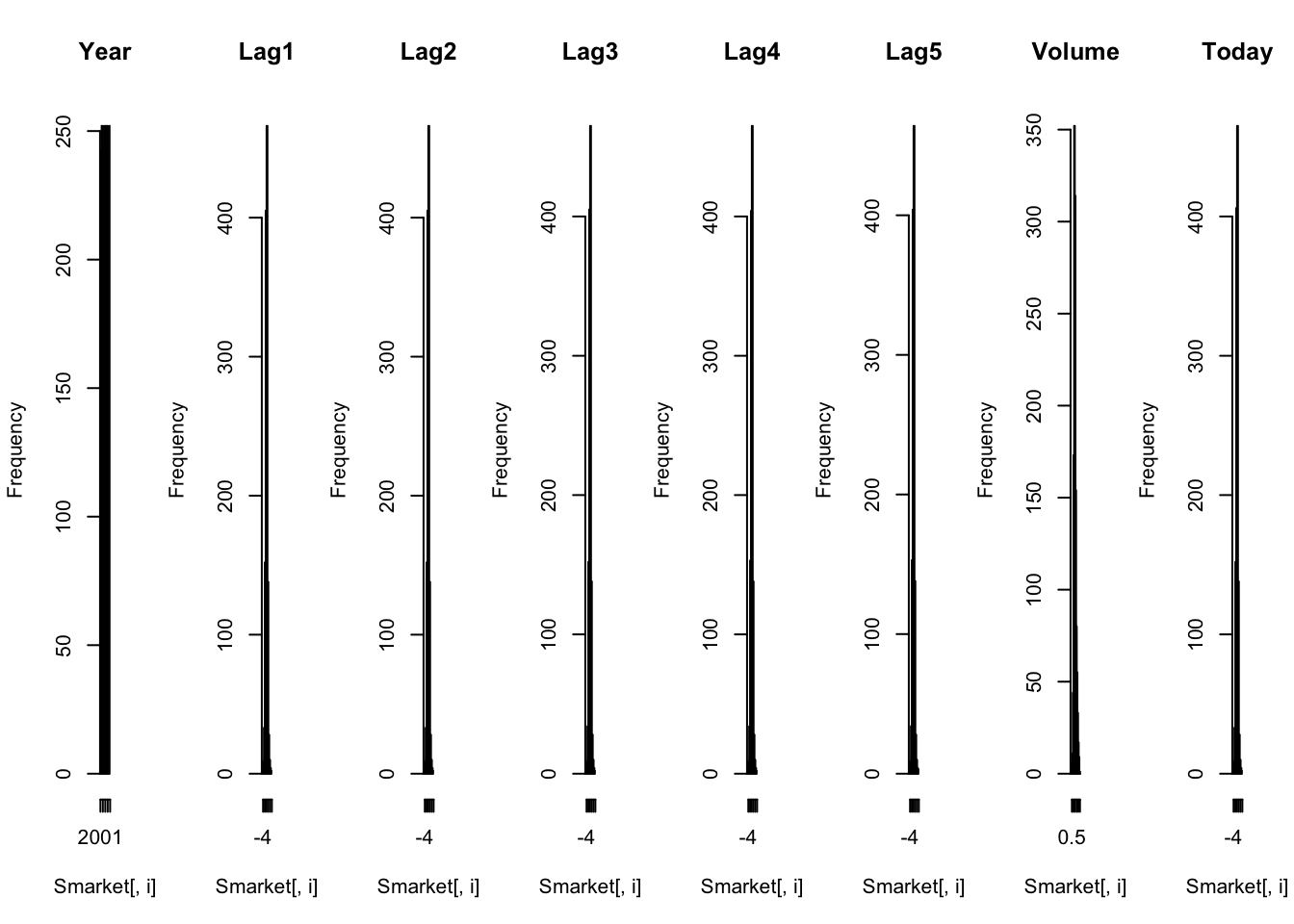It's extremely hard to see, but most of the variables show a Gaussian or double Gaussian distribution.

You can look at the distribution of the data a different way using box and whisker plots. The box captures the middle 50% of the data, the line shows the median and the whiskers of the plots show the reasonable extent of data. Any dots outside the whiskers are good candidates for outliers.

par(mfrow=c(1,8))
for(i in 1:8) {
boxplot(Smarket[,i], main=names(Smarket)[i])
}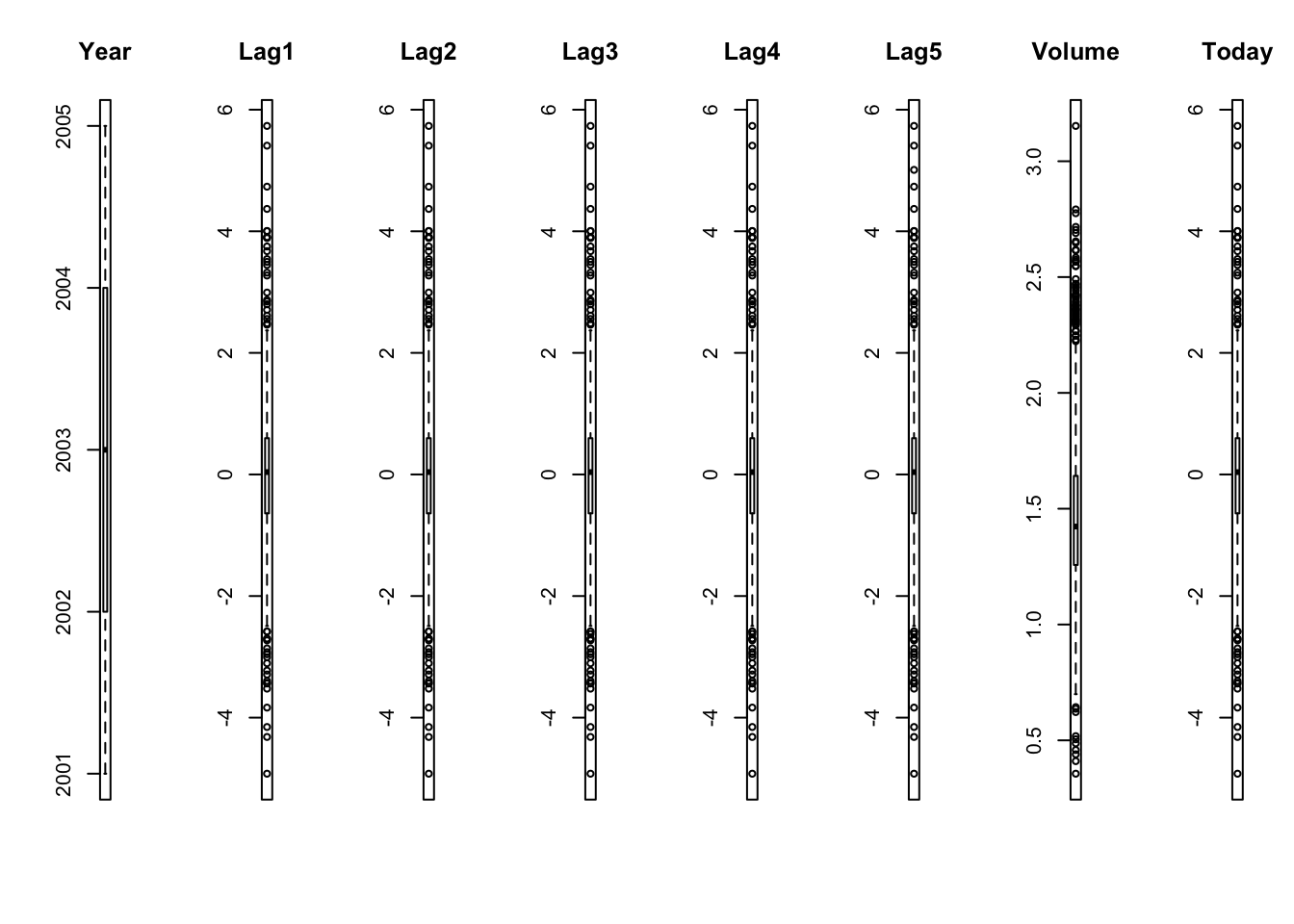You can see that the Lags and Today all has a similar range. Otherwise, there's no sign of any outliers.

Missing data have have a big impact on modeling. Thus, you can use a missing plot to get a quick idea of the amount of missing data in the dataset. The x-axis shows attributes and the y-axis shows instances. Horizontal lines indicate missing data for an instance, vertical blocks represent missing data for an attribute.

library(Amelia)
library(mlbench)
missmap(Smarket, col=c("blue", "red"), legend=FALSE)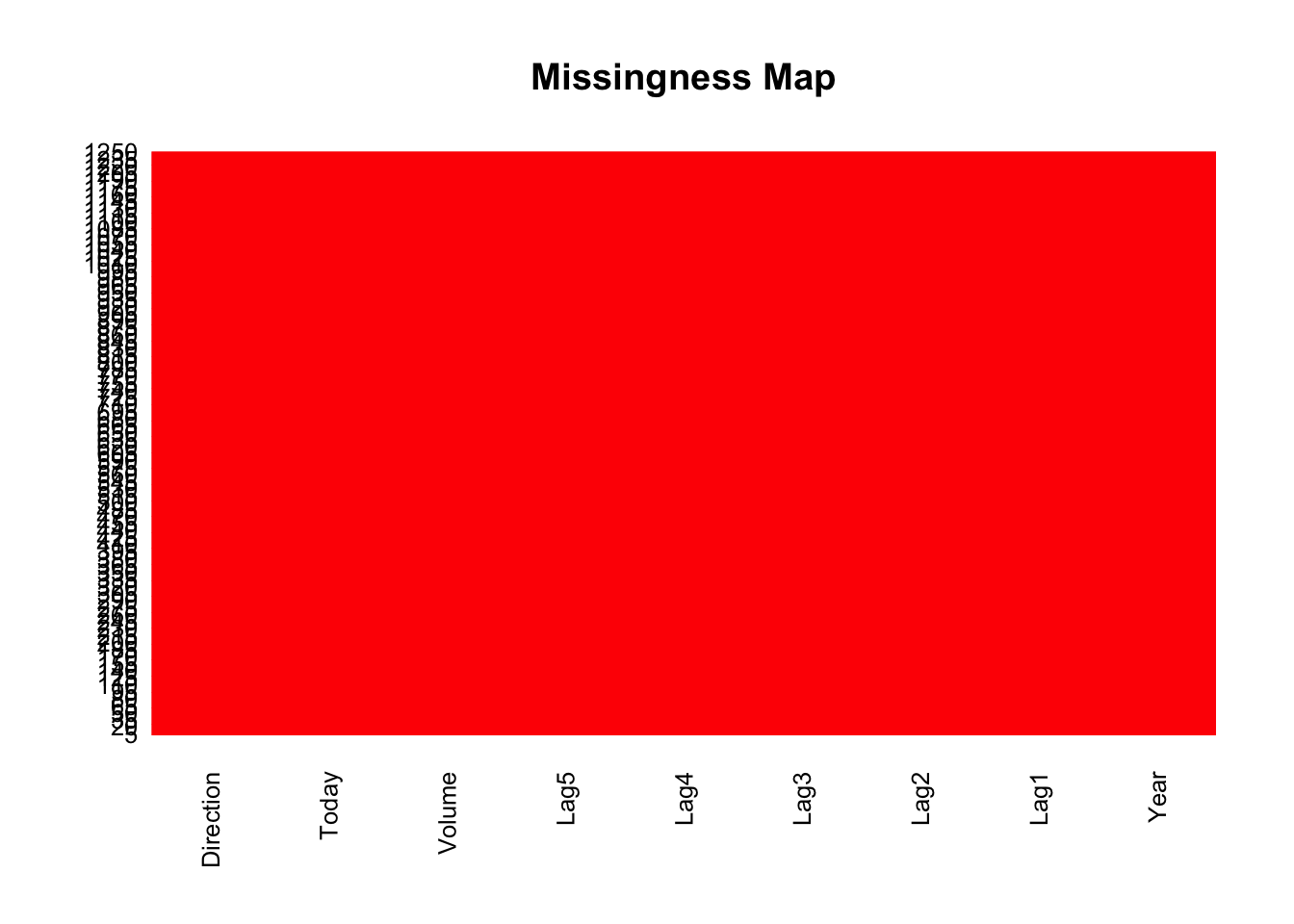Well, lucky for me! No missing data in this dataset!

Let's start calculating the correlation between each pair of numeric variables. These pair-wise correlations can be plotted in a correlation matrix plot to given an idea of which variables change together.

library(corrplot)
correlations <- cor(Smarket[,1:8])
corrplot(correlations, method="circle")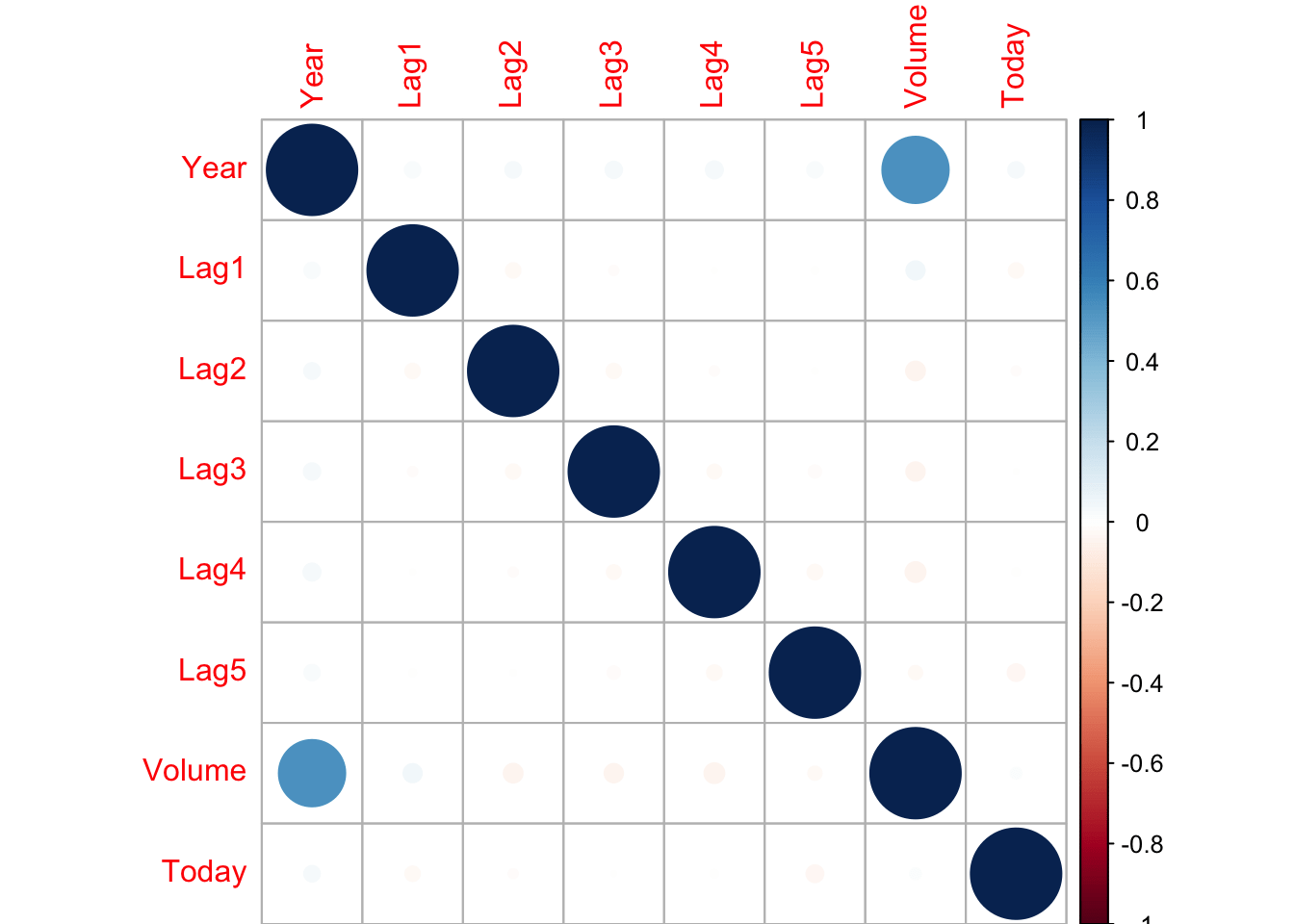A dot-representation was used where blue represents positive correlation and red negative. The larger the dot the larger the correlation. You can see that the matrix is symmetrical and that the diagonal are perfectly positively correlated because it shows the correlation of each variable with itself. Unfortunately, none of the variables are correlated with one another.

Let's make a plot of the data. There's a pairs() function which plots the variables in Smarket into a scatterplot matrix. In this case, Direction, your binary response, is the color indicator:

pairs(Smarket, col=Smarket$Direction)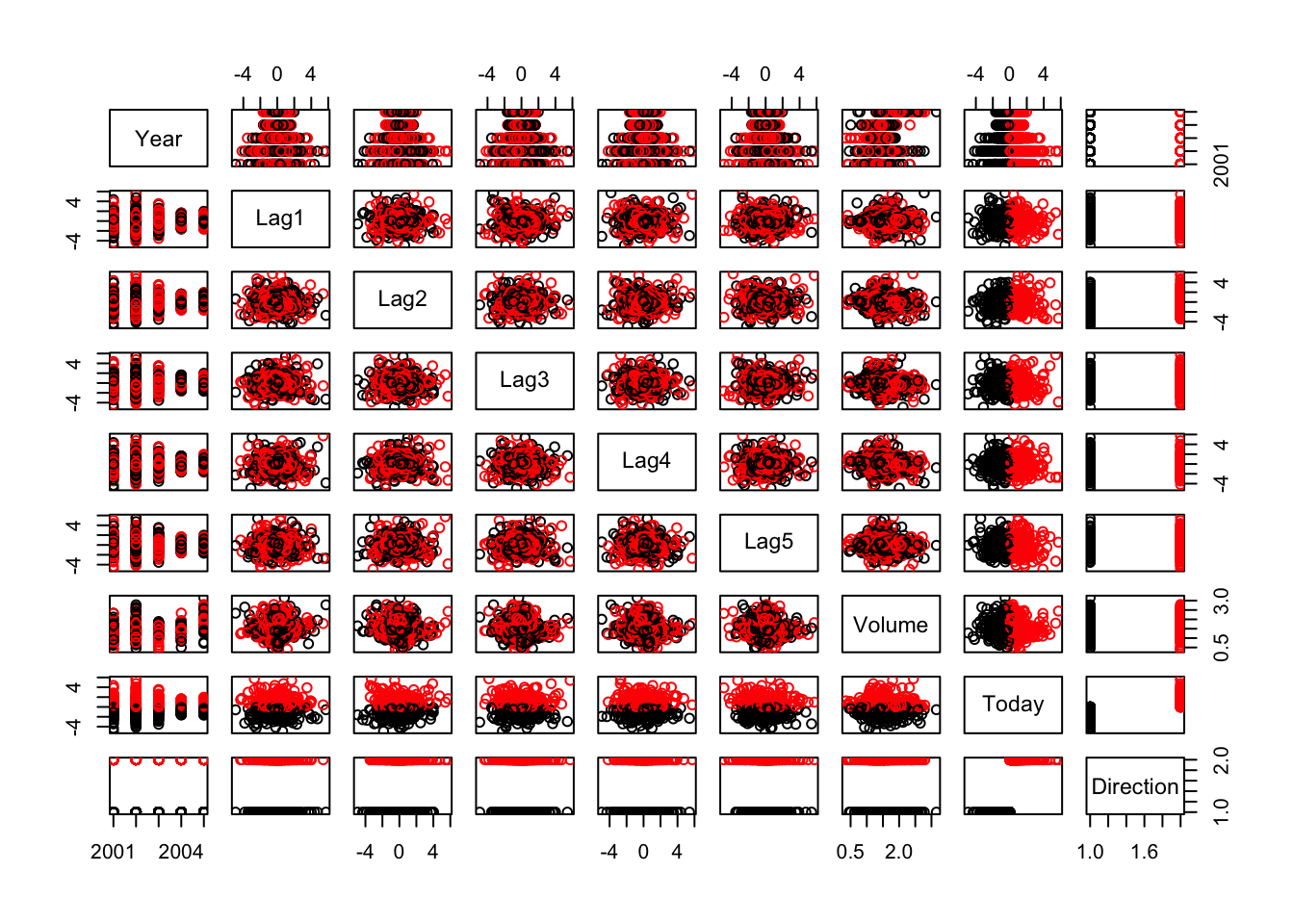It looks like there's not much correlation going on here. The class variable is derived from the variable Today, so Up and Down seems to make a division. Other than that, there's not much going on. Let's take a look at the density distribution of each variable broken down by Direction value. Like the scatterplot matrix above, the density plot by Direction can help see the separation of Up and Down. It can also help to understand the overlap in Direction values for a variable. library(caret) x <- Smarket[,1:8] y <- Smarket[,9] scales <- list(x=list(relation="free"), y=list(relation="free")) featurePlot(x=x, y=y, plot="density", scales=scales)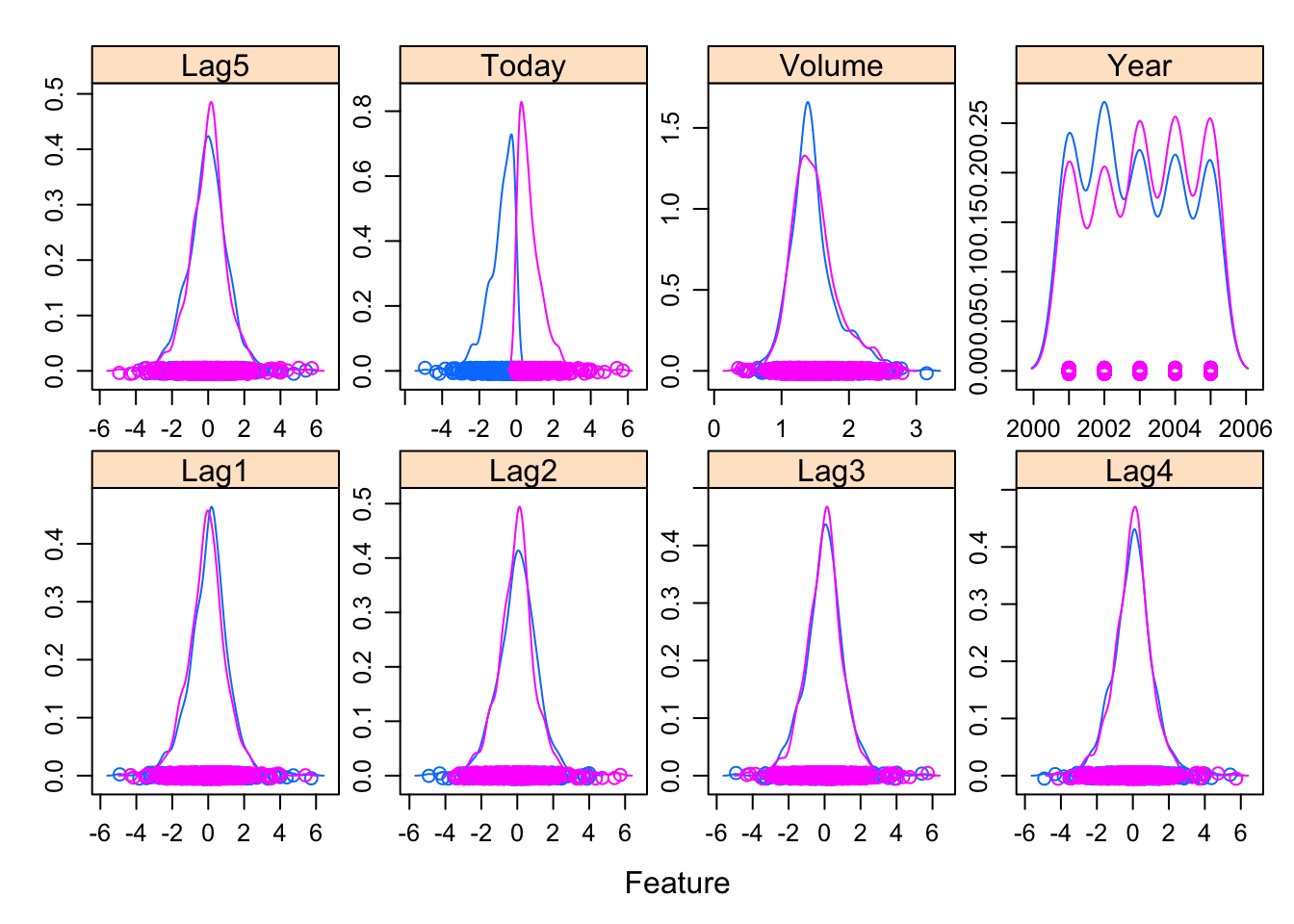You can see that the Direction values overlap for all of these variables, meaning that it's hard to predict Up or Down based on just one or two variables. ### Building Logistic Regression Model Now you call glm.fit() function. The first argument that you pass to this function is an R formula. In this case, the formula indicates that Direction is the response, while the Lag and Volume variables are the predictors. As you saw in the introduction, glm is generally used to fit generalized linear models. However, in this case, you need to make it clear that you want to fit a logistic regression model. You resolve this by setting the family argument to binomial. This way, you tell glm() to put fit a logistic regression model instead of one of the many other models that can be fit to the glm. # Logistics Regression glm.fit <- glm(Direction ~ Lag1 + Lag2 + Lag3 + Lag4 + Lag5 + Volume, data = Smarket, family = binomial)  Next, you can do a summary(), which tells you something about the fit: summary(glm.fit) ## ## Call: ## glm(formula = Direction ~ Lag1 + Lag2 + Lag3 + Lag4 + Lag5 + ## Volume, family = binomial, data = Smarket) ## ## Deviance Residuals: ## Min 1Q Median 3Q Max ## -1.446 -1.203 1.065 1.145 1.326 ## ## Coefficients: ## Estimate Std. Error z value Pr(>|z|) ## (Intercept) -0.126000 0.240736 -0.523 0.601 ## Lag1 -0.073074 0.050167 -1.457 0.145 ## Lag2 -0.042301 0.050086 -0.845 0.398 ## Lag3 0.011085 0.049939 0.222 0.824 ## Lag4 0.009359 0.049974 0.187 0.851 ## Lag5 0.010313 0.049511 0.208 0.835 ## Volume 0.135441 0.158360 0.855 0.392 ## ## (Dispersion parameter for binomial family taken to be 1) ## ## Null deviance: 1731.2 on 1249 degrees of freedom ## Residual deviance: 1727.6 on 1243 degrees of freedom ## AIC: 1741.6 ## ## Number of Fisher Scoring iterations: 3  As you can see, summary() returns the estimate, standard errors, z-score, and p-values on each of the coefficients. Look like none of the coefficients are significant here. It also gives you the null deviance (the deviance just for the mean) and the residual deviance (the deviance for the model with all the predictors). There's a very small difference between the 2, along with 6 degrees of freedom. You assign the result of predict() of glm.fit() to glm.probs, with type equals to response. This will make predictions on the training data that you use to fit the model and give me a vector of fitted probabilities. You look at the first 5 probabilities and they are very close to 50%: glm.probs <- predict(glm.fit,type = "response") glm.probs[1:5] ## 1 2 3 4 5 ## 0.5070841 0.4814679 0.4811388 0.5152224 0.5107812  Now I am going to make a prediction of whether the market will be up or down based on the lags and other predictors. In particular, I'll turn the probabilities into classifications by thresholding at 0.5. In order to do so, I use an ifelse() command. glm.pred <- ifelse(glm.probs > 0.5, "Up", "Down")  glm.pred is a vector of trues and falses. If glm.probs is bigger than 0.5, glm.pred calls "Up"; otherwise, it calls "False". Here, you attach the data frame Smarket and make a table of glm.pred, which is the ups and downs from the previous direction. You also take the mean of those. attach(Smarket) table(glm.pred,Direction) ## Direction ## glm.pred Down Up ## Down 145 141 ## Up 457 507 mean(glm.pred == Direction) ##  0.5216  From the table, instances on the diagonals are where you get the correct classification, and off the diagonals are where you make mistake. Looks like you made a lot of mistakes. The mean gives a proportion of 0.52. ### Creating Training and Test Samples How can you do better? Dividing the data up into a training set and a test set is a good strategy. # Make training and test set train = Year<2005 glm.fit <- glm(Direction ~ Lag1 + Lag2 + Lag3 + Lag4 + Lag5 + Volume, data = Smarket, family = binomial, subset = train) glm.probs <- predict(glm.fit, newdata = Smarket[!train,], type = "response") glm.pred <- ifelse(glm.probs > 0.5, "Up", "Down")  Let's look at this code chunk in detail: • train is equal to the year less than 2005. For all the year less than 2005, you'll get a true; otherwise, I'll get a false. • You then refit the model with glm.fit(), except that the subset is equal to 'train', which means that it fits to just the data in year less than 2005. • You then use the predict() function again for glm.probs to predict on the remaining data in year greater or equal to 2005. For the new data, You give it Smarket, indexed by !train (!train is true if the year is greater or equal to 2005). You set type to "response" to predict the probabilities. • Finally, you use the ifelse() function again for glm.pred to make Up and Down variable. You now make a new variable to store a new subset for the test data and call it Direction.2005. The response variable is still Direction. You make a table and compute the mean on this new test set: Direction.2005 = Smarket$Direction[!train]
table(glm.pred, Direction.2005)

##         Direction.2005
## glm.pred Down Up
##     Down   77 97
##     Up     34 44

mean(glm.pred == Direction.2005)

##  0.4801587


Ha, you did worse than the previous case. How could this happen?

### Solving Overfitting

Well, you might have overfitted the data. In order to fix this, you're going to fit a smaller model and use Lag1, Lag2, Lag3 as the predictors, thereby leaving out all other variables. The rest of the code is the same.

# Fit a smaller model
glm.fit = glm(Direction ~ Lag1 + Lag2 + Lag3, data = Smarket, family = binomial, subset = train)
glm.probs = predict(glm.fit, newdata = Smarket[!train,], type = "response")
glm.pred = ifelse(glm.probs > 0.5, "Up", "Down")
table(glm.pred, Direction.2005)

##         Direction.2005
## glm.pred Down  Up
##     Down   39  31
##     Up     72 110

mean(glm.pred == Direction.2005)

##  0.5912698


Well, you got a classification rate of 59%, not too bad. Using the smaller model appears to perform better.

Lastly, you will do a summary() of glm.fit to see if there are any signficant changes.

summary(glm.fit)

##
## Call:
## glm(formula = Direction ~ Lag1 + Lag2 + Lag3, family = binomial,
##     data = Smarket, subset = train)
##
## Deviance Residuals:
##    Min      1Q  Median      3Q     Max
## -1.338  -1.189   1.072   1.163   1.335
##
## Coefficients:
##              Estimate Std. Error z value Pr(>|z|)
## (Intercept)  0.032230   0.063377   0.509    0.611
## Lag1        -0.055523   0.051709  -1.074    0.283
## Lag2        -0.044300   0.051674  -0.857    0.391
## Lag3         0.008815   0.051495   0.171    0.864
##
## (Dispersion parameter for binomial family taken to be 1)
##
##     Null deviance: 1383.3  on 997  degrees of freedom
## Residual deviance: 1381.4  on 994  degrees of freedom
## AIC: 1389.4
##
## Number of Fisher Scoring iterations: 3


Nothing became significant, at least the P-values are better, indicating an increase in prediction of performance.

## Conclusion

So that's the end of this R tutorial on building logistic regression models using the glm() function and setting family to binomial. glm() does not assume a linear relationship between dependent and independent variables. However, it assumes a linear relationship between link function and independent variables in logit model I hope you have learned something valuable!